Question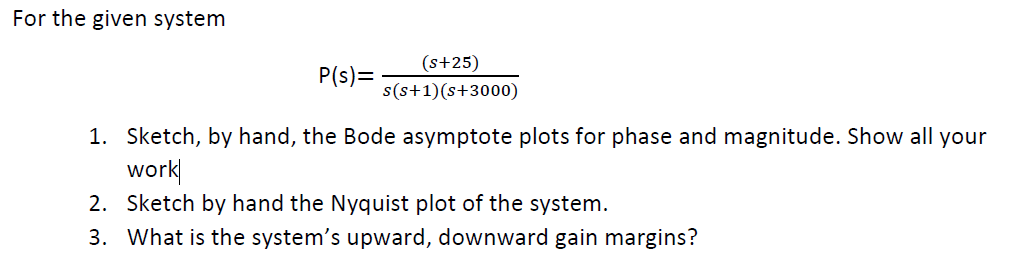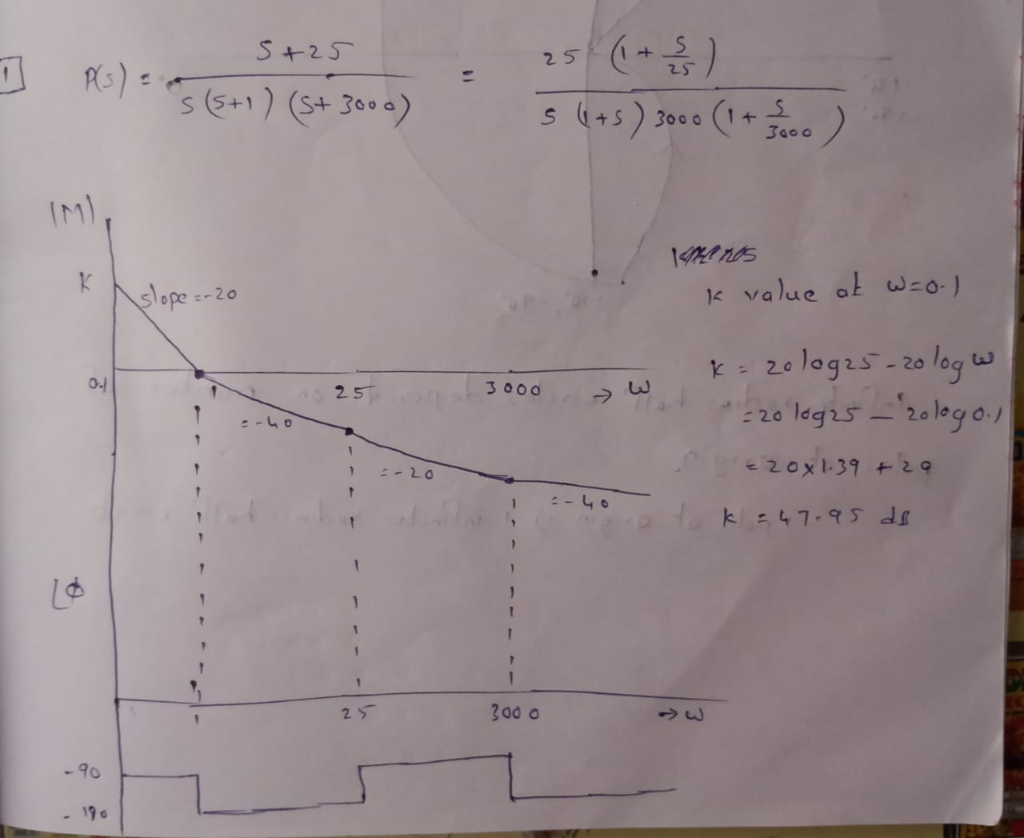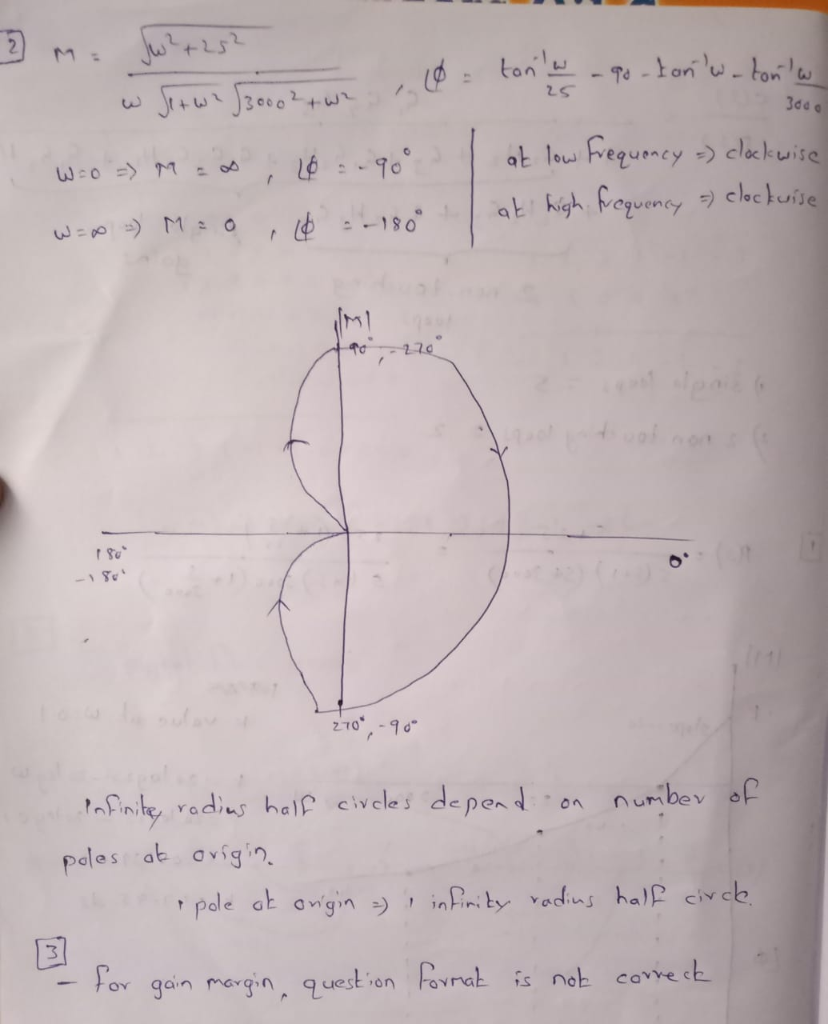#### Earn Coins

Coins can be redeemed for fabulous gifts.

Similar Homework Help Questions
• ### Please explain how to sketch by hand the bode asymptote plots for magnititude and phase of:...

Please explain how to sketch by hand the bode asymptote plots for magnititude and phase of: P(s) = (s+35)/[(s)(s+3.5)(s+3500)]

• ### For the following closed-loop transfer functions, sketch the bode plots (magnitude and phase), id...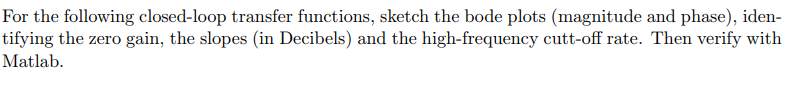For the following closed-loop transfer functions, sketch the bode plots (magnitude and phase), iden- tifying the zero gain, the slopes (in Decibels) and the high-frequency cutt-off rate. Then verify with Matlab (6) wn = 1, 〈 0.0.1, and 0.707. (8) Assuming the system of Problem 6 above, and an input of r(t) = 30sin(1000 t), use your bode plot to obtain the steady-state response For the following closed-loop transfer functions, sketch the bode plots (magnitude and phase), iden- tifying the...

• ### Sketch the approximate Bode magnitude and phase plots for the following transfer functions by hand. a....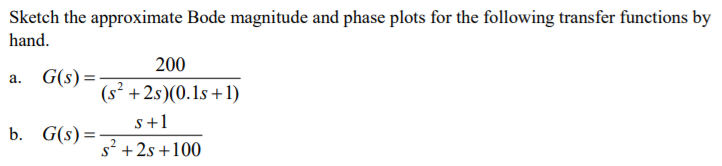Sketch the approximate Bode magnitude and phase plots for the following transfer functions by hand. a. G(s) b. G(s)- 200 (s2 +2s)(0.1s +1) s+1 s2 +2s +100

• ### i) Draw the Bode plots (hand sketch, magnitude and phase!) for the following transfer function. Plot...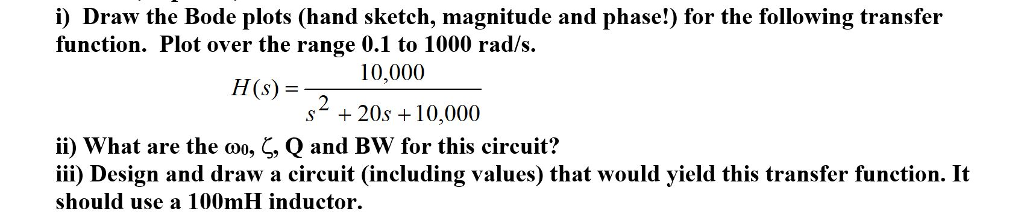i) Draw the Bode plots (hand sketch, magnitude and phase!) for the following transfer function. Plot over the range 0.1 to 1000 rad/s HS 10,000 (s) = s* + 20s 10,000 ii) what are the Q and Bw for this circuit? iii) Design and draw a circuit (including values) that would yield this transfer function. It should use a 100mH inductor , , Qano

• ### 3 Bode sketch 40 pts Sketch the Bode asymptotic magnitude and asymptotic phase plots for G(s)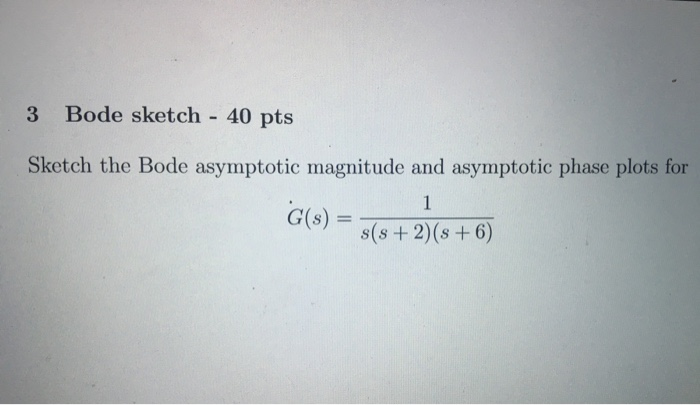3 Bode sketch 40 pts Sketch the Bode asymptotic magnitude and asymptotic phase plots for G(s)

• ### Problem 5: For the following transfer functions, sketch the bode asymptotic magnitude and phase p...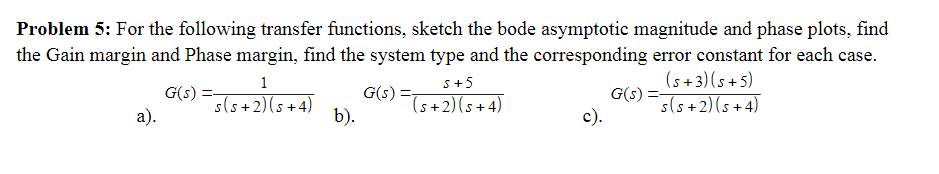Problem 5: For the following transfer functions, sketch the bode asymptotic magnitude and phase plots, find the Gain margin and Phase margin, find the system type and the corresponding error constant for each case. G(A) (s +3)(s +5) s(s +2) (s+4) S+5 2)b). Problem 5: For the following transfer functions, sketch the bode asymptotic magnitude and phase plots, find the Gain margin and Phase margin, find the system type and the corresponding error constant for each case. G(A) (s +3)(s...

• ### A unity feedback control system has the open loop TF as

A unity feedback control system has the open loop TF as: $$G(s)=\frac{K(s+a+1)(s+b)}{s(s+a)(s+a+2)}$$a) Find analytical expressions for the magnitude and phase response for $$\mathrm{G}(\mathrm{s}) .\left[K=K_{1}\right]$$b) Make a plot of the log-magnitude and the phase, using log-frequency in rad/s as the ordinate. $$\left[K=K_{1}\right]$$c) Sketch the Bode asymptotic magnitude and asymptotic phase plots. $$\left[K=K_{1}\right]$$d) Compare the results from $$(a),(b)$$, and $$(c) .\left[K=K_{1}\right]$$e) Using the Nyquist criterion, find out if system is stable. Show your steps. $$\left[K=K_{1}\right]$$f) Using the Nyquist criterion, find the range...

• ### For the following transfer function, sketch approximate straight-line Bode plots, including magnitude and phase plots. Show...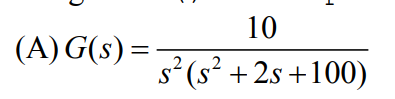For the following transfer function, sketch approximate straight-line Bode plots, including magnitude and phase plots. Show all steps clearly 10 4 (A)G(s)-7 s (s 2s +100)

• ### Please plot on semi-log scale for both magnitude and phase separately B. Sketch the Bode plots...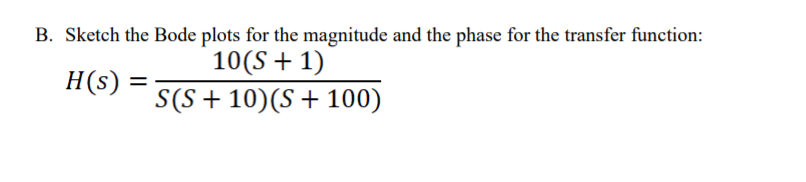Please plot on semi-log scale for both magnitude and phase separately B. Sketch the Bode plots for the magnitude and the phase for the transfer function: 10(S + 1) H(S) = S(S + 10)(8 + 100)

• ### Bode Plots Sketch the Bode plot magnitude and phase for each of the three open-loop transfer...

Bode Plots Sketch the Bode plot magnitude and phase for each of the three open-loop transfer functions listed below. Verify your results using the bode m function in MATLAB.(a) $$G(s)=\frac{100}{s(0.1 s+1)(0.01 s+1)}$$(b) $$G(s)=\frac{1}{(s+1)^{2}\left(s^{2}+s+9\right)}$$(c) $$G(s)=\frac{16000 s}{(s+1)(s+100)\left(s^{2}+5 s+1600\right)}$$